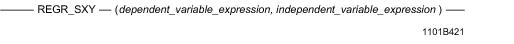# 15.00 - REGR_SXY - Teradata Database

## Teradata Database SQL Functions, Operators, Expressions, and Predicates

Product
Release Number
15.00
Content Type
Programming Reference
Publication ID
B035-1145-015K
Language
English (United States)

## REGR_SXY

### Purpose

Returns the sum of the products of the independent_variable_expression and the dependent_variable_expression for all non‑null data pairs of the dependent and independent variable arguments.

### Syntaxwhere:

 Syntax element … Specifies … dependent_variable_expression the dependent variable for the regression. A dependent variable is something that is measured in response to a treatment. The expression cannot contain any ordered analytical or aggregate functions. independent_variable_expression the independent variable for the regression. An independent variable is a treatment: something that is varied under your control to test the behavior of another variable. The expression cannot contain any ordered analytical or aggregate functions.

### ANSI Compliance

This is ANSI SQL:2011 compliant.

### Setting Up Axes for Plotting

If you export the data for plotting, define the y-axis (ordinate) as the dependent variable and the x-axis (abscissa) as the independent variable.

### Combination With Other Functions

REGR_SXY can be combined with any of the ordered analytical functions in a SELECT list, QUALIFY clause, or ORDER BY clause. For more information on ordered analytical functions, see Chapter 22: “Ordered Analytical / Window Aggregate Functions.”

REGR_SXY cannot be combined with aggregate functions within the same SELECT list, QUALIFY clause, or ORDER BY clause.

### Computation

The equation for computing REGR_SXY is defined as follows:This variable … Represents … x independent_variable_expression x is the independent, or predictor, variable expression. y dependent_variable_expression y is the dependent, or response, variable expression. n COUNT(x,y)

When there are fewer than two non-null data point pairs in the data used for the computation, then REGR_SXY returns NULL.

Division by zero results in NULL rather than an error.

### Result Type and Attributes

The data type, format, and title for REGR_SXY(y, x) are as follows.

Data type: REAL

• If the operand is character, the format is the default format for FLOAT.
• If the operand is numeric, date, or interval, the format is the same format as y.
• If the operand is UDT, the format is the format for the data type to which the UDT is implicitly cast.
• For information on the default format of data types, see “Data Type Formats and Format Phrases” in SQL Data Types and Literals.

### Support for UDTs

By default, Teradata Database performs implicit type conversion on UDT arguments that have implicit casts that cast between the UDTs and any of the following predefined types:

• Numeric
• Character
• DATE
• Interval
• To define an implicit cast for a UDT, use the CREATE CAST statement and specify the AS ASSIGNMENT clause. For more information on CREATE CAST, see SQL Data Definition Language.

Implicit type conversion of UDTs for system operators and functions, including REGR_SXY, is a Teradata extension to the ANSI SQL standard. To disable this extension, set the DisableUDTImplCastForSysFuncOp field of the DBS Control Record to TRUE. For details, see Utilities: Volume 1 (A-K).

For more information on implicit type conversion of UDTs, see Chapter 13: “Data Type Conversions.”

### REGR_SXY Window Function

For the REGR_SXY window function that performs a group, cumulative, or moving computation, see “Window Aggregate Functions” on page 984.

### Example

This example is based the following regrtbl data. Nulls are indicated by the QUESTION MARK character.

`c1   height   weight`
`--   ------   ------`
` 1       60       84`
` 2       62       95`
` 3       64      140`
` 4       66      155`
` 5       68      119`
` 6       70      175`
` 7       72      145`
` 8       74      197`
` 9       76      150`
`10       76      ?`
`11       ?       150`
`12       ?       ?`
` `

The following SELECT statement returns the sum of products of height and weight where neither height nor weight is null.

`   SELECT REGR_SXY(weight,height) `
`   FROM regrtbl;`
`   Regr_Sxy(weight,height)`
`   -----------------------`
`                      1200`